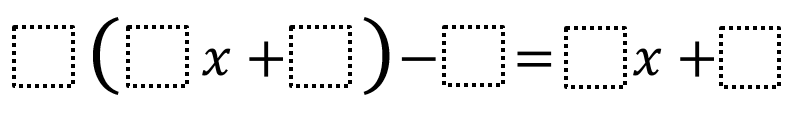# Equivalent Expressions 2

Directions: Using the digits 1 to 9 at most one time each, fill in the boxes to create two equivalent expressions.### Hint

How does subtracting a number affect the left side of the equation?

Number of Unique Solutions: 26
1: 2(3x + 4) – 1 = 6x + 7
2: 2(3x + 4) – 7 = 6x + 1
3: 2(3x + 5) – 1 = 6x + 9
4: 2(3x + 5) – 9 = 6x + 1
5: 2(3x + 7) – 5 = 6x + 9
6: 2(3x + 7) – 9 = 6x + 5
7: 2(3x + 8) – 7 = 6x + 9
8: 2(3x + 8) – 9 = 6x + 7
9: 2(4x + 3) – 1 = 8x + 5
10: 2(4x + 3) – 5 = 8x + 1
11: 2(4x + 5) – 1 = 8x + 9
12: 2(4x + 5) – 3 = 8x + 7
13: 2(4x + 5) – 7 = 8x + 3
14: 2(4x + 5) – 9 = 8x + 1
15: 2(4x + 6) – 3 = 8x + 9
16: 2(4x + 6) – 5 = 8x + 7
17: 2(4x + 6) – 7 = 8x + 5
18: 2(4x + 6) – 9 = 8x + 3
19: 2(4x + 7) – 5 = 8x + 9
20: 2(4x + 7) – 9 = 8x + 5
21: 3(2x + 4) – 5 = 6x + 7
22: 3(2x + 4) – 7 = 6x + 5
23: 3(2x + 5) – 7 = 6x + 8
24: 3(2x + 5) – 8 = 6x + 7
25: 4(2x + 3) – 5 = 8x + 7
26: 4(2x + 3) – 7 = 8x + 5

Source: Will Case

## Greatest Common Factor

Directions: Using the digits 0 to 9 at most one time each, place a digit …

1.What I think is really cool about these problems about equivalent expressions is that even though there are multiple answers (and in this case, MANY answers), there are restrictions about where certain numbers can go in the expression – like, all of the samples posted have either a 2 or a 3 in the factor outside of the parentheses.

2.2(3x + 6) – 4 = 6x + 8

3.4.